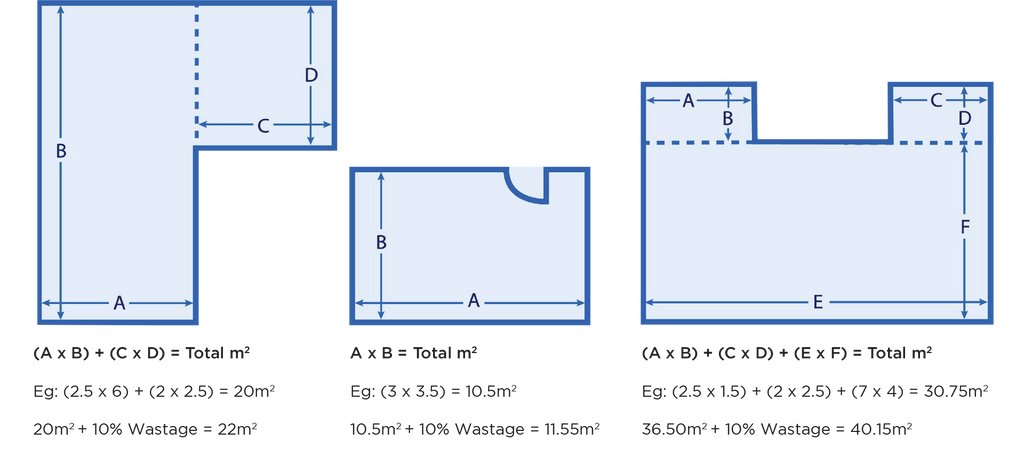Working out the quantity of flooring that you need is really dependant on the layout of your room.

Rectangular rooms can be calculated easily by multiplying the length by width. If your room is irregularly shaped, you need to divide your room into separate smaller areas, adding these areas together to calculate the total square meters. Add an additional 10% to your square meter total for wastage.+
=
x
=